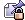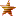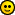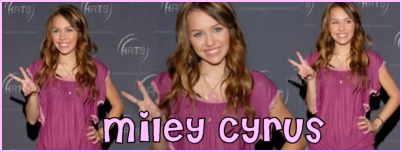Xtra Smileys [Open]
 Glamurous and Sassy Chickz Read House Rules Before Posting!
September 24, 2020, 11:17:37 pm
 News: Welcome to Glamurous And Sassy Chikz.. Hope you will enjoy here!!# Symbol Codes[MERGED]

 Pages:Author Topic: Symbol Codes[MERGED]  (Read 738 times)
Web Master Cutie
Girly ChickKarma: +54/-0
Posts: 237Web Master« on: February 16, 2008, 11:16:19 pm »

I created this thread so that members will know the codes for those musical notes and others..
(this is from 1-100)

alt+1=☺
alt+2=☻
alt+3=♥
alt+4=♦
alt+5=♣
alt+6=♠
alt+7=
alt+8=◘
alt+9=○
alt+1+0=◙
alt+1+1=♂
alt+1+2=♀
alt+1+3=♪
alt+1+4=♫
alt+1+5=☼
alt+1+6=►
alt+1+7=◄
alt+1+8=↕
alt+1+9=‼
alt+2+0=¶
alt+2+1=§
alt+2+2=▬
alt+2+3=↨
alt+2+4=↑
alt+2+5=↓
alt+2+6=→
alt+2+7=←
alt+2+8=∟
alt+2+9=↔
alt+3+0=▲
alt+3+1=▼
alt+3+2=space
alt+3+3=!
alt+3+4="
alt+3+5=#
alt+3+6=\$
alt+3+7=%
alt+3+8=&
alt+3+9='
alt+4+0=(
alt+4+1=)
alt+4+2=*
alt+4+3=+
alt+4+4=,
alt+4+5=-
alt+4+6=.
alt+4+7=/
alt+4+8=0
alt+4+9=1
alt+5+0=2
alt+5+1=3
alt+5+2=4
alt+5+3=5
alt+5+4=6
alt+5+5=7
alt+5+6=8
alt+5+7=9
alt+5+8=:
alt+5+9=;
alt+6+0=<
alt+6+1= =
alt+6+2=>
alt+6+3=?
alt+6+4=@
alt+6+5=A
alt+6+6=B
alt+6+7=C
alt+6+8=D
alt+6+9=E
alt+7+0=F
alt+7+1=G
alt+7+2=H
alt+7+3=I
alt+7+4=J
alt+7+5=K
alt+7+6=L
alt+7+7=M
alt+7+8=N
alt+7+9=O
alt+8+0=P
alt+8+1=Q
alt+8+2=R
alt+8+3=S
alt+8+4=T
alt+8+5=U
alt+8+6=V
alt+8+7=W
alt+8+8=X
alt+8+9=Y
alt+9+0=Z
alt+9+1=[
alt+9+2=\
alt+9+3=]
alt+9+4=^
alt+9+5=_
alt+9+6=`
alt+9+7=a
alt+9+8=b
alt+9+9=c
alt+1+0+0=d

Bonus:
alt+1+5+9=
alt+1+6+0=á
I will lock this thread so that we can avoid posting spam stuff and going off topic...

If you want to ask a question just post it right away!!Report SpamLoggedThanks Snowflake078. Read The House Rules. No spamming and No Double-Posting!!

 Pages: